Lately I’ve been looking to explore time-series modeling and to get more practice with manipulating data in R. Luckily, a dataset on Kaggle provided me the opportunity to do both of these things. Attempting to predict future sale volume of items in stores (from 1-C russian store sales dataset) gave me the chance to apply the theoretical knowledge I’ve been studying of time-series analysis (ARIMA in particular). Additionally, I was able to get more comfortable with dplyr and lubridate in the process.

The variable of interest here was a daily count of sales for each item-store combo, equating to over 2 million rows of data. The process I took was to:

-aggregate sales data into monthly counts (this is what Kaggle contest is looking for)

-separate each store-item combo out into its own time-series.

-Explore how appropriate an ARIMA model is and if differentiation is called for

-Find a model which could generalize the data for each combo effectively

-Run the function to predict next months sales for the store-item combo.

Overall, this route of pretty effective, although final results show an ARIMA model may have been too complex for what was needed. A later run trial of exponential smoothing beat my best ARIMA model by about .15 root mean square error. Not too surprising as ARIMa models should have at least 40 points in the time-series, while these each had only 34. Additionally, many of the time-series were quite sparse, which is not the best input for ARIMA modeling.

Regardless, this was great practice, gave me a better understanding of working with time-series, and in particular how to judge stationarity and appropriateness for ARIMA.

NOTE: many of the lines are commented out.. Most of these were excluded from my final knit as compuation times were a bit excessive for some of the loops and functions I ended up using.

knitr::opts_chunk$set(echo = TRUE) library(tidyverse) ## -- Attaching packages -------------------------------------------------------- tidyverse 1.2.1 -- ## v ggplot2 2.2.1 v purrr 0.2.4 ## v tibble 1.4.2 v dplyr 0.7.4 ## v tidyr 0.8.0 v stringr 1.2.0 ## v readr 1.1.1 v forcats 0.3.0 ## -- Conflicts ----------------------------------------------------------- tidyverse_conflicts() -- ## x dplyr::filter() masks stats::filter() ## x dplyr::lag() masks stats::lag() library(xts) ## Loading required package: zoo ## ## Attaching package: 'zoo' ## The following objects are masked from 'package:base': ## ## as.Date, as.Date.numeric ## ## Attaching package: 'xts' ## The following objects are masked from 'package:dplyr': ## ## first, last library(lubridate) ## ## Attaching package: 'lubridate' ## The following object is masked from 'package:base': ## ## date library(tseries) ## Time Series Analysis in R Exploring daily sales data of software firm 1C. This dataset was obtained from Kaggle and is used for the final project of the Coursera “How to win a data science competition” coutse. NOTE: I did not take this course! First to import the data and see how it looks: #train data train <- read.csv('data/train.csv', stringsAsFactors = FALSE) #test data & Kaggle sample sub test <- read.csv('data/test.csv') sample_sub <- read.csv('data/sample_submission.csv') #additional data included items <- read.csv('data/items.csv') categories <- read.csv('data/item_categories.csv') shops <- read.csv("data/shops.csv") ## Describe the datasets Initial exploration to check data types, sizes, etc. dim(train) ##  2935849 6 head(train) ## date date_block_num shop_id item_id item_price item_cnt_day ## 1 02.01.2013 0 59 22154 999.00 1 ## 2 03.01.2013 0 25 2552 899.00 1 ## 3 05.01.2013 0 25 2552 899.00 -1 ## 4 06.01.2013 0 25 2554 1709.05 1 ## 5 15.01.2013 0 25 2555 1099.00 1 ## 6 10.01.2013 0 25 2564 349.00 1 dim(test) ##  214200 3 head(test) ## ID shop_id item_id ## 1 0 5 5037 ## 2 1 5 5320 ## 3 2 5 5233 ## 4 3 5 5232 ## 5 4 5 5268 ## 6 5 5 5039 head(sample_sub) ## ID item_cnt_month ## 1 0 0.5 ## 2 1 0.5 ## 3 2 0.5 ## 4 3 0.5 ## 5 4 0.5 ## 6 5 0.5 So, looks as if we’re to predict the ‘item_cnt_month’ based upon ‘shop_id’ and ‘item_id’.. A few things this informs us: Need to craft a ‘daily sales’item_cnt_day’ varible in the train set into a monthly sale count to have an appropriate dependant variable for model fitting. This should be done by -Aggregate daily sales into monthy, split by shop_id and item_id. Once this is done, we have two other variables of interest in the train: -date : we’ll perform some time-series analysis here -price: I’m, sure there is some coreelation between this and monthly sales. So, first step is to create the monthly sales variable in train per shop/item. Then we can explore trends and seasonality in the time-series. #First set year variable and edit typeas train$year <- substr(train$date, 7, 10) train$year <- as.numeric(train$year) train$month <- substr(train$date, 4, 5) train$month <- as.numeric(train$month) train$date <- as.Date(train$date, "%d.%m.%Y") sapply(train, class) ## date date_block_num shop_id item_id item_price ## "Date" "integer" "integer" "integer" "numeric" ## item_cnt_day year month ## "numeric" "numeric" "numeric" Should have done this first probably :/ lets check for missing values real quick. sapply(train, function(x) sum(is.na(x))) ## date date_block_num shop_id item_id item_price ## 0 0 0 0 0 ## item_cnt_day year month ## 0 0 0 Love Kaggle for that. Lets divert from the train for a second and add the next block count, year, and month to the test set. We know it’s the next year/month occuring right after the end of the train set, which is october 2015. #date <- as.Date("01.11.2015", "%d.%m.%Y") #for (i in 1:nrow(test)){ # test[i,4] = 35 # test[i, 5] = 2015 # test[i, 6] = 11 #} #colnames(test)[4:6] <- c('date_block_num', 'year', 'month') Lets also split out the needed data into a train set which accounts for monthly sales rather than daily. #train$my <- floor_date(train$date, "month") df_train <- train %>% group_by(date_block_num, shop_id, item_id, year, month) %>% summarize(item_cnt_month = sum(item_cnt_day))  ## Basic Median fit I fit fit and modeled the data based upon only the mean monthly sales per item per shop. Any NA’s are filled with the median of monthly item sales throughout entire dataset… I call this the basic af model, and it’s a nice one to set as a comparable to the future time-series.. #basic <- df_train %>% group_by(shop_id, item_id) %>% # summarize(mean_count = median(item_cnt_month)) #overall_mean <- mean(basic$mean_count)

#basic_test <- test %>% select(ID, shop_id, item_id)

#basic_test <- merge(x = basic_test, y = basic, by = c("shop_id", "item_id"), all.x = TRUE)
#basic_test$mean_count[is.na(basic_test$mean_count)] <- overall_mean

#preds = basic_test$mean_count #sample_sub$item_cnt_month = preds

#write.csv(sample_sub, file = "D://projects/kaggle/time_series/basic_af.csv", row.names = #FALSE)

So back to work on the time series.. lets figure out how to set it up by exploring one or two specific shop-item combos.

ts_train <- train %>% group_by(date_block_num, shop_id, item_id) %>%
summarize(item_cnt_month = sum(item_cnt_day))# %>%
#mutate(ts = xts(item_cnt_month, order.by = as.Date(ts_train$date))) ### TRIAL HERE ts_test <- subset(ts_train, ts_train$shop_id == 10 & ts_train$item_id == 1871) ts <- rnorm(34, m = 0, sd = 0) ts <- ts(ts, start = 2013, frequency = 12) for (i in 1:34){ if(i %in% ts_test$date_block_num){
j <- which(ts_test$date_block_num == i) num <- ts_test[j,4] ts[i] <- as.numeric(num) } } plot(ts) abline(reg = lm(ts~time(ts)))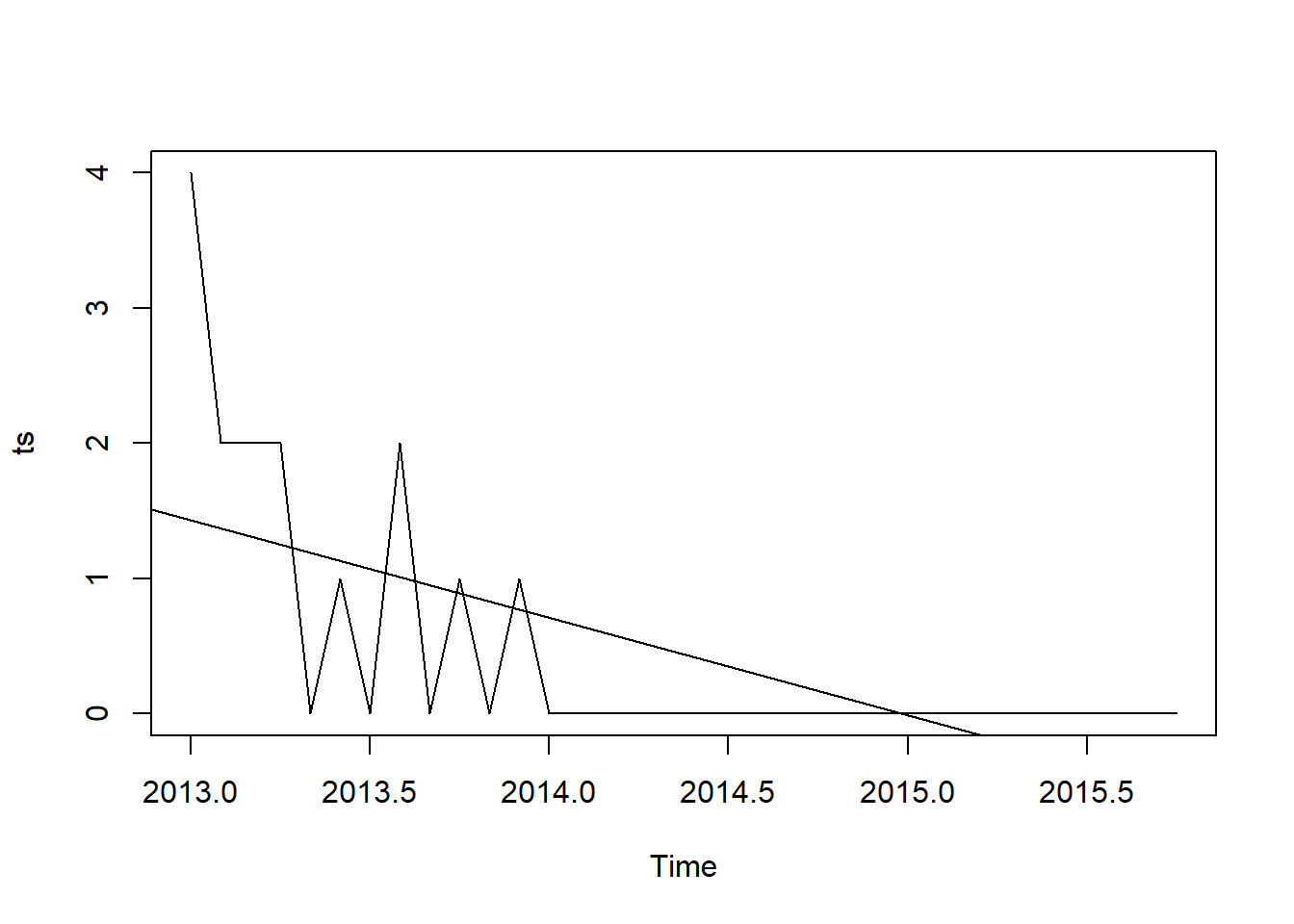### END HERE I’m going to focus on checking the time-series for trend/seasonality first. Performing ARIMA requires stationary data. lets make sure the cycle is correct. cycle(ts) ## Jan Feb Mar Apr May Jun Jul Aug Sep Oct Nov Dec ## 2013 1 2 3 4 5 6 7 8 9 10 11 12 ## 2014 1 2 3 4 5 6 7 8 9 10 11 12 ## 2015 1 2 3 4 5 6 7 8 9 10 boxplot(ts~cycle(ts))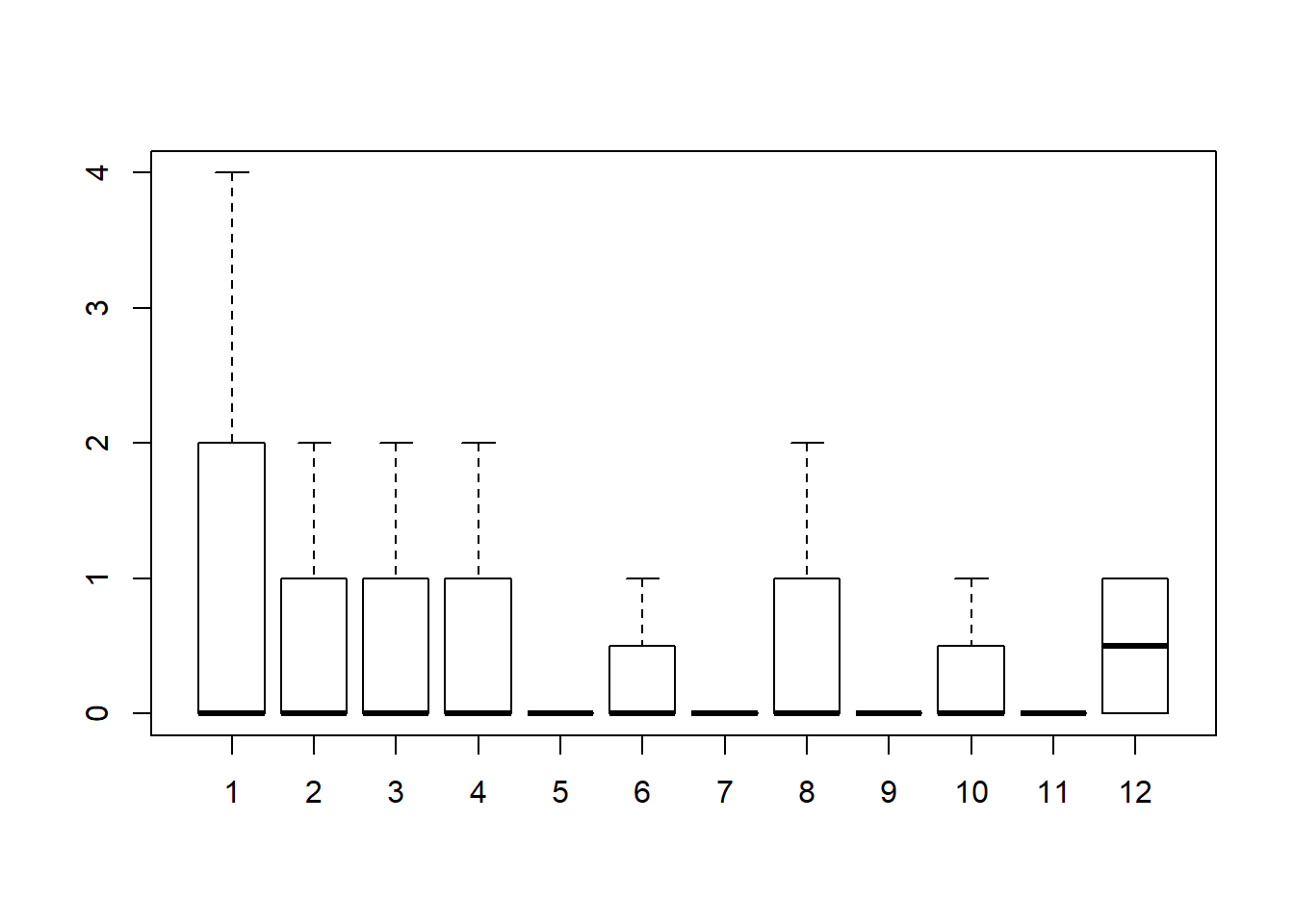Hmmm I’d argue that this specific shop-item combo doesn’t quite need a model to accuracy predict… It’s zero’d out the past two years and every month’s median is 0. Variance remains the same so no need to log.. additional, log doesn’t act nicely with 0… Our sale items are dropping, but not quite negative infinity. adf.test(ts, alternative = "stationary", k = 0) ## Warning in adf.test(ts, alternative = "stationary", k = 0): p-value smaller ## than printed p-value ## ## Augmented Dickey-Fuller Test ## ## data: ts ## Dickey-Fuller = -6.3398, Lag order = 0, p-value = 0.01 ## alternative hypothesis: stationary adf.test(diff(ts), alternative="stationary", k=0) ## Warning in adf.test(diff(ts), alternative = "stationary", k = 0): p-value ## smaller than printed p-value ## ## Augmented Dickey-Fuller Test ## ## data: diff(ts) ## Dickey-Fuller = -15.55, Lag order = 0, p-value = 0.01 ## alternative hypothesis: stationary acf(ts)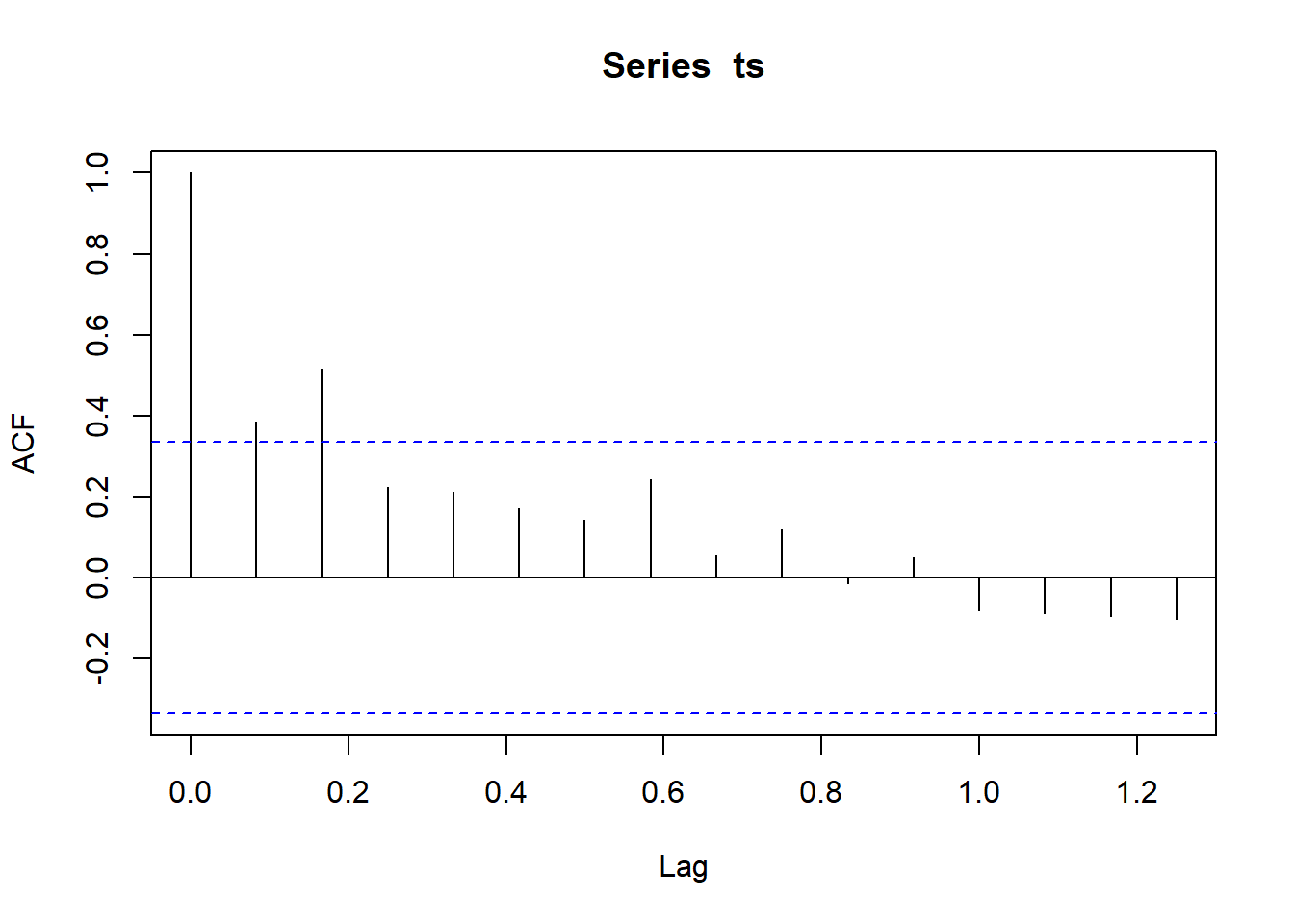acf(diff(ts))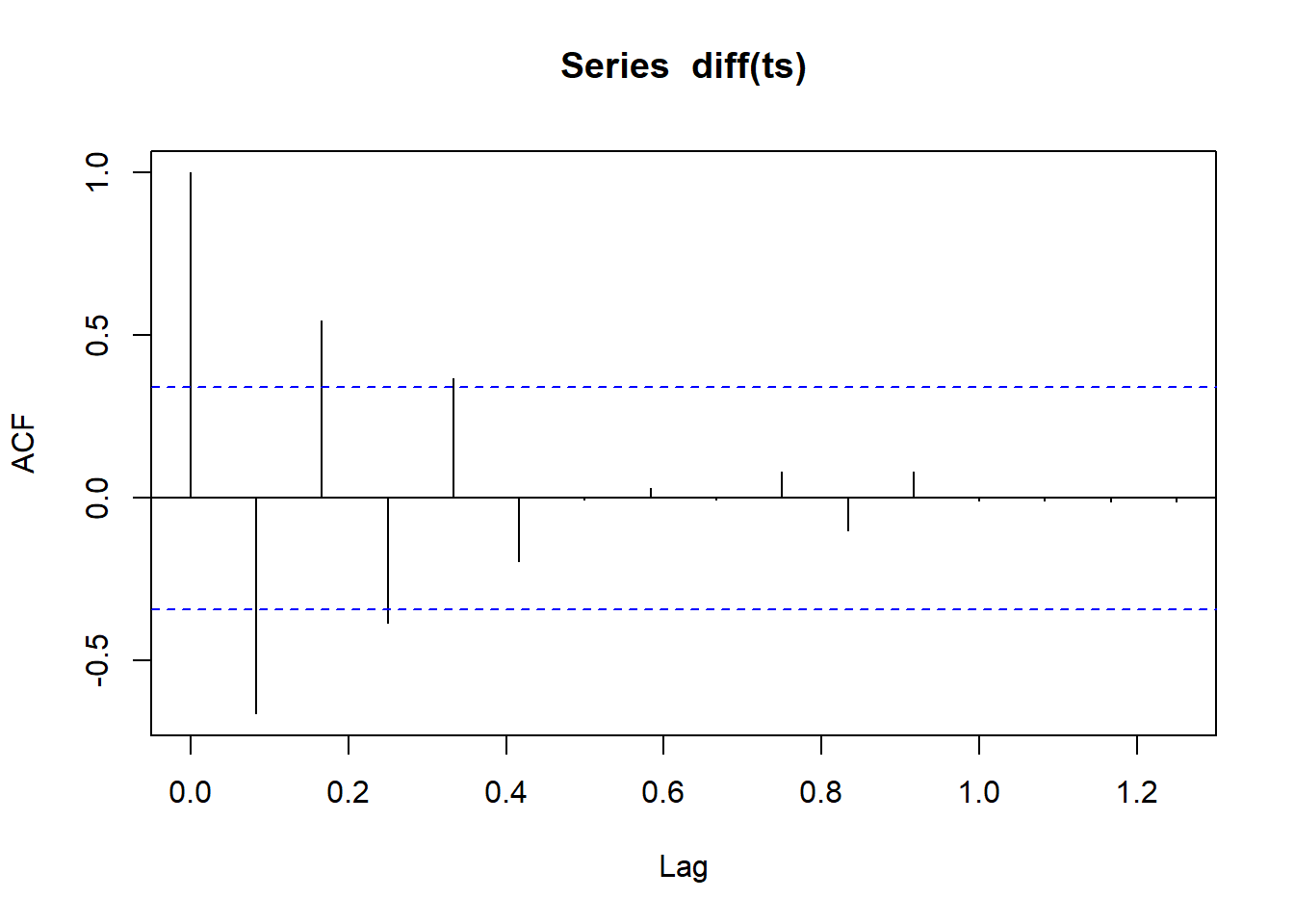I’m voting on the differentiated version. lets check PACF as well pacf(diff(ts))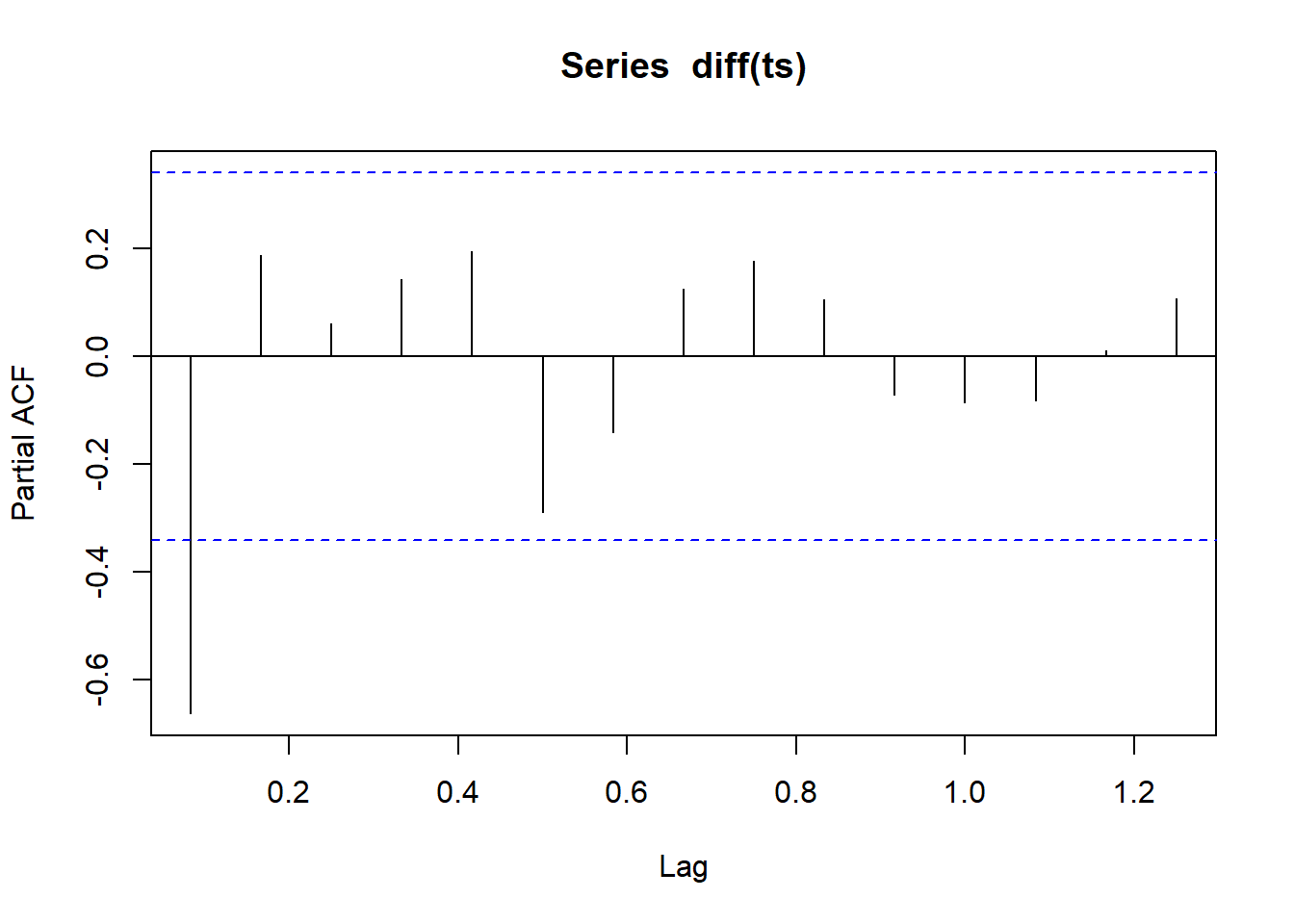Based on the plots, I think it looks like we have an ARIMA model setup of a 1 in auto-regession and 1 or 2 in moving average.. Lets go with the 2 here so AR, I, MA ->(1,1,2) fit112 <- arima(ts, order = c(1, 1, 2)) pred112 <- predict(fit112) ts.plot(ts, pred112$pred, lty = c(1,3))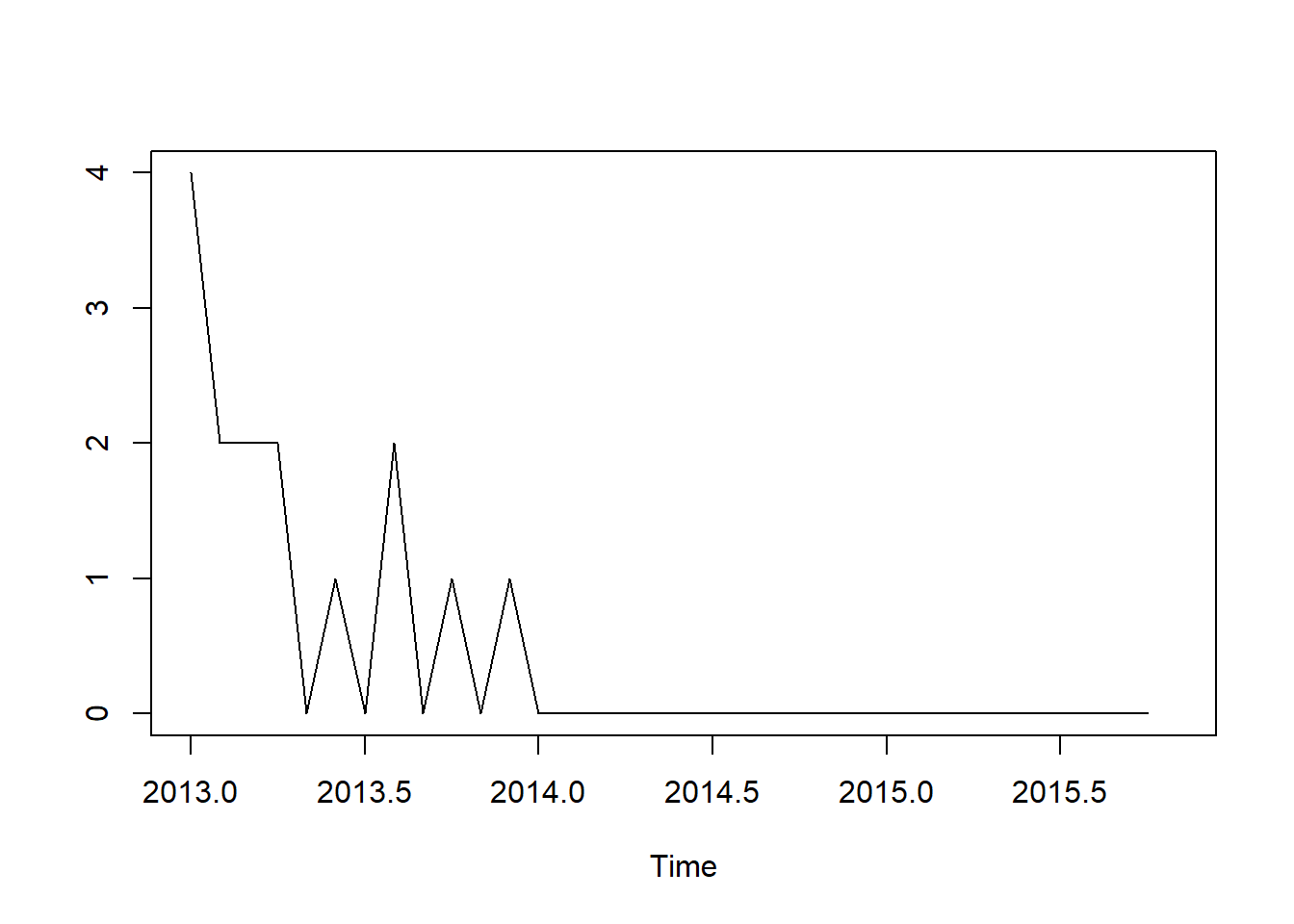pred112$pred ## Nov ## 2015 -5.093003e-09 Aright.. its looks good.. an estimate of very very very close to 0. Lets find a shop-item with more sales and see how this same setup does. #ts_test <- subset(ts_train, ts_train$shop_id == 31 & ts_train$item_id == 20949) #ts <- rnorm(34, m = 0, sd = 0) #ts <- ts(ts, start = 2013, frequency = 12) #for (i in 1:34){ # if(i %in% ts_test$date_block_num){
#    j <- which(ts_test$date_block_num == i) # num <- ts_test[j,4] # ts[i] <- as.numeric(num) # } #} #plot(ts) #abline(reg = lm(ts~time(ts))) #boxplot(ts~cycle(ts)) #adf.test(ts, alternative = "stationary", k = 0) #adf.test(diff(ts), alternative="stationary", k=0) #acf(diff(ts)) #pacf(diff(ts)) #fit011 <- arima(ts, order = c(0, 1, 1)) #pred011 <- predict(fit011) #ts.plot(ts, pred011$pred, lty = c(1,3))

#pred011$pred Alright, hard to tell is a 0 sounds right for this item.. It has no sales in the store for the past 3 months, so it may have been taken off the shelves? lets find one with sales in the latest few months to test the model with: ts_test <- subset(ts_train, ts_train$shop_id == 48 & ts_train$item_id == 20949) ts <- rnorm(34, m = 0, sd = 0) ts <- ts(ts, start = 2013, frequency = 12) for (i in 1:34){ if(i %in% ts_test$date_block_num){
j <- which(ts_test$date_block_num == i) num <- ts_test[j,4] ts[i+1] <- as.numeric(num) } } plot(ts) abline(reg = lm(ts~time(ts)))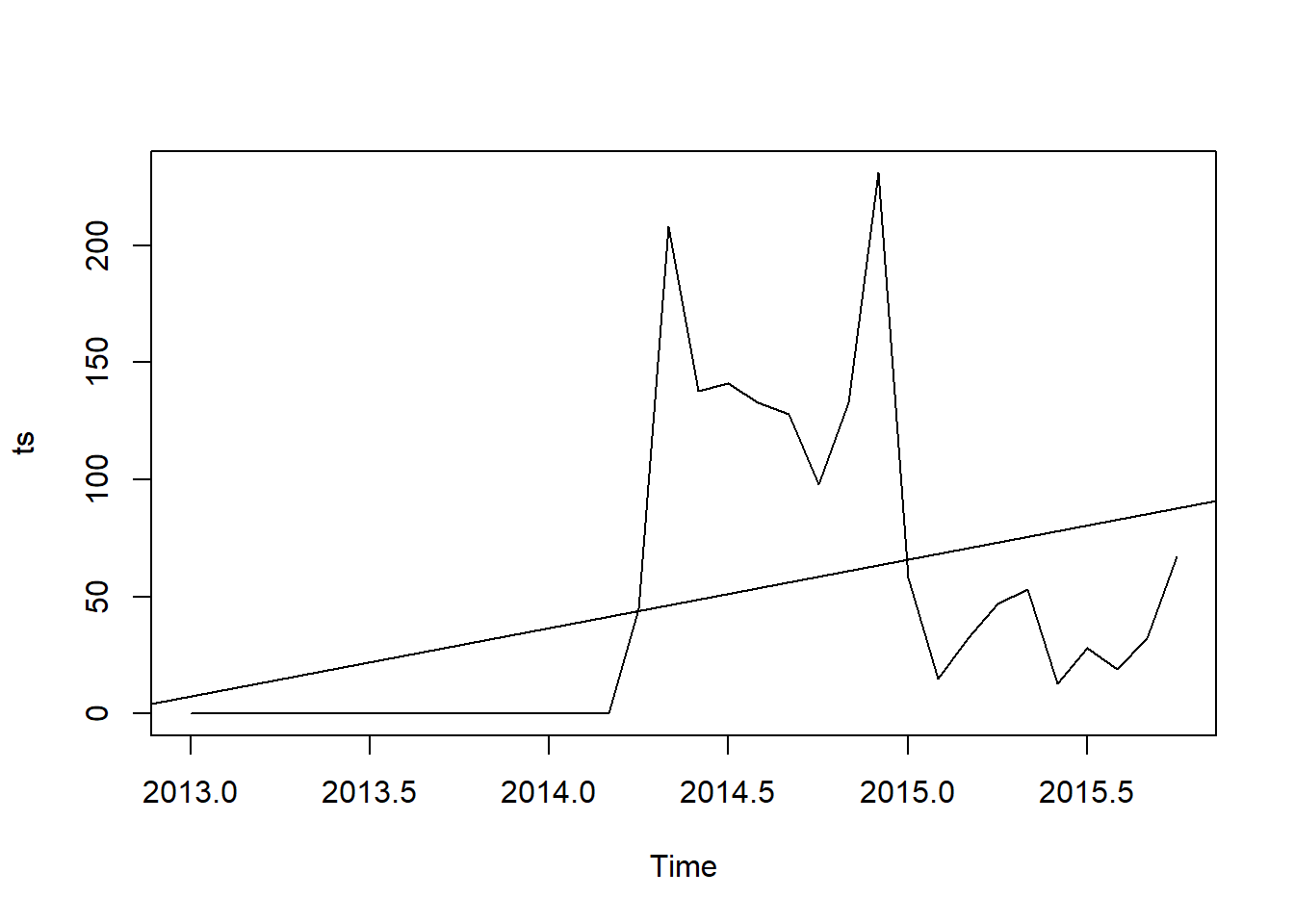boxplot(ts~cycle(ts))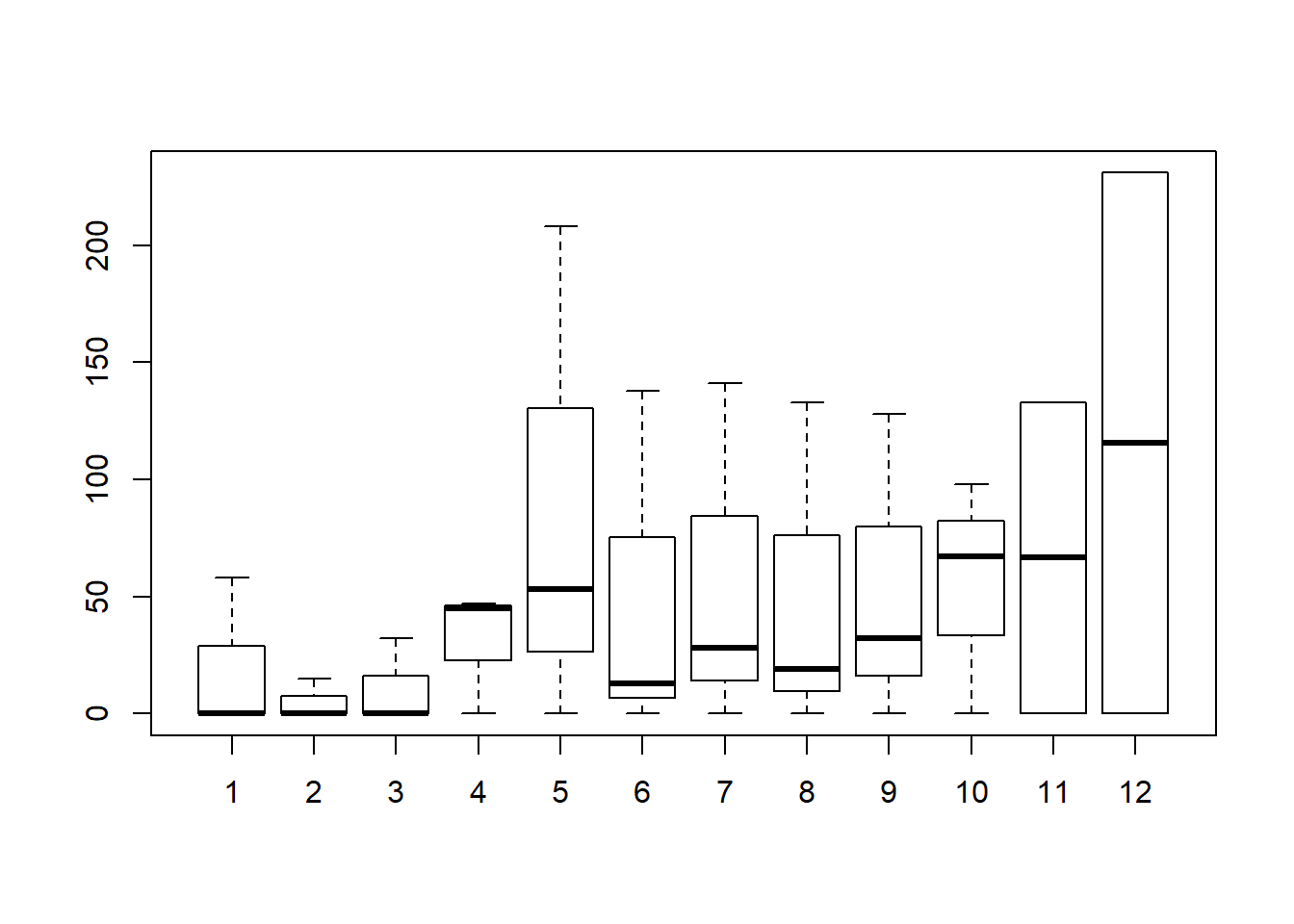adf.test(ts, alternative = "stationary", k = 0) ## ## Augmented Dickey-Fuller Test ## ## data: ts ## Dickey-Fuller = -2.4661, Lag order = 0, p-value = 0.3924 ## alternative hypothesis: stationary adf.test(diff(ts), alternative="stationary", k=0) ## ## Augmented Dickey-Fuller Test ## ## data: diff(ts) ## Dickey-Fuller = -6.2593, Lag order = 0, p-value = 0.01 ## alternative hypothesis: stationary acf(diff(ts))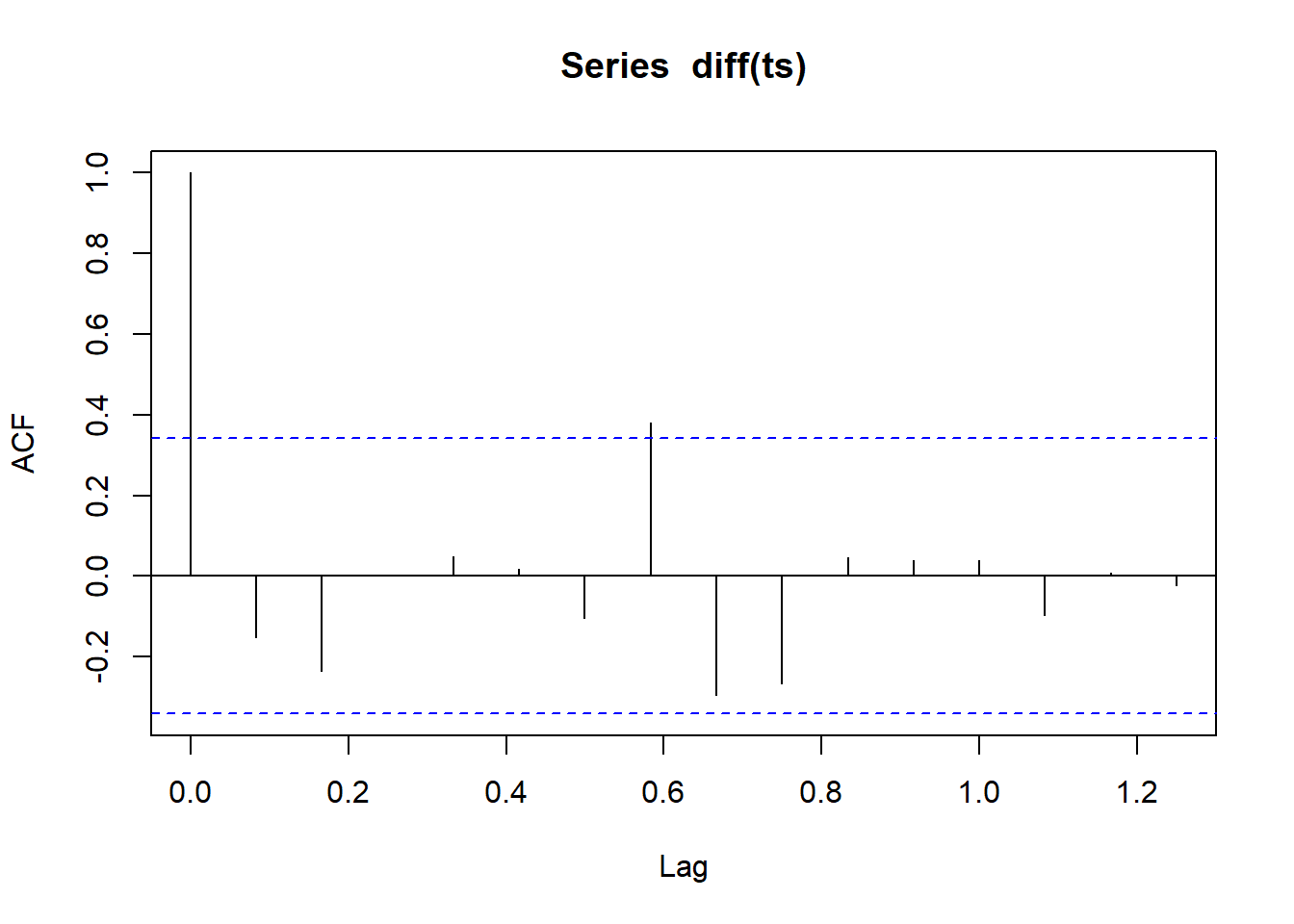pacf(diff(ts))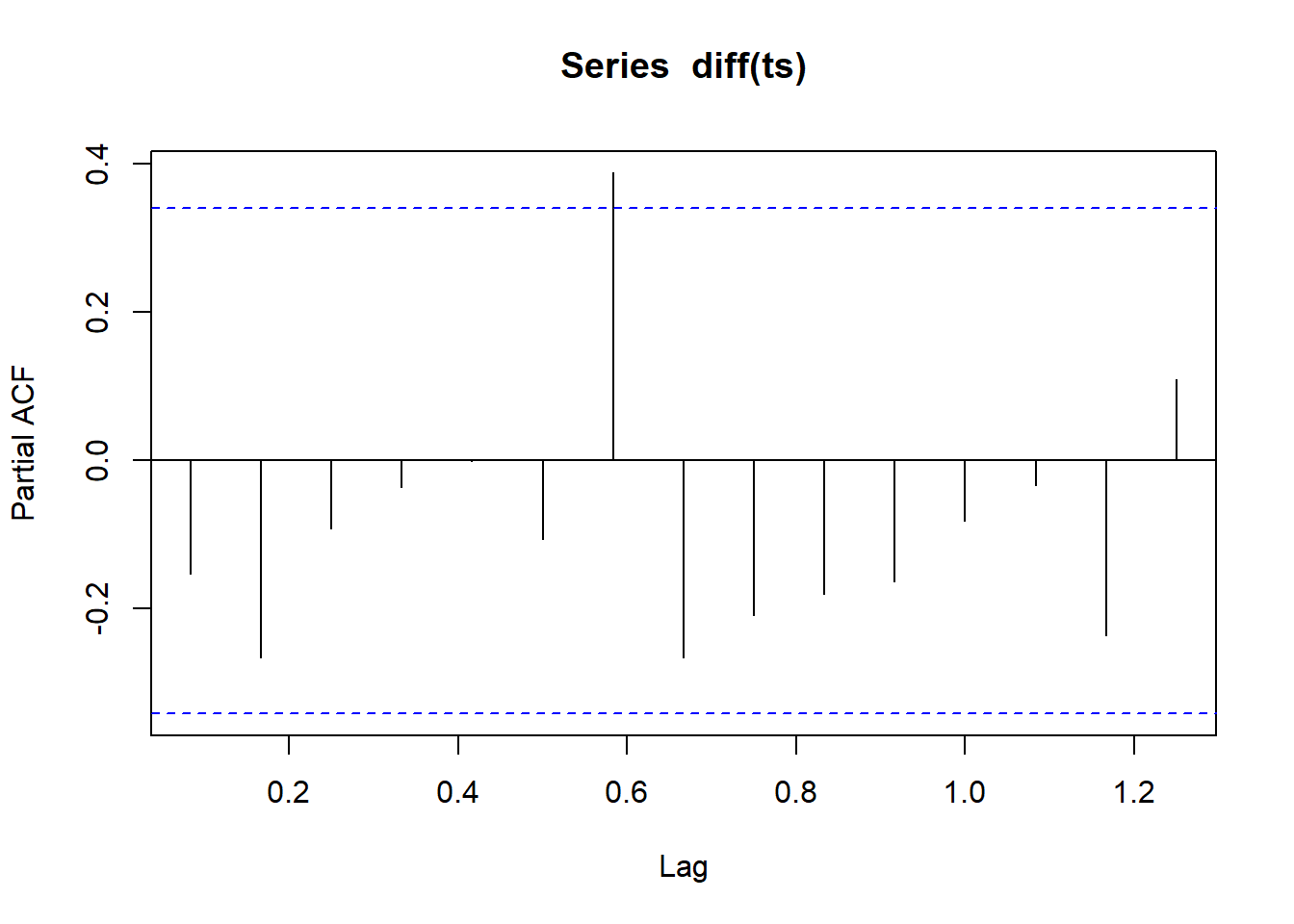fit112 <- arima(ts, order = c(1, 1, 2)) pred112 <- predict(fit112) ts.plot(ts, pred112$pred, lty = c(1,3))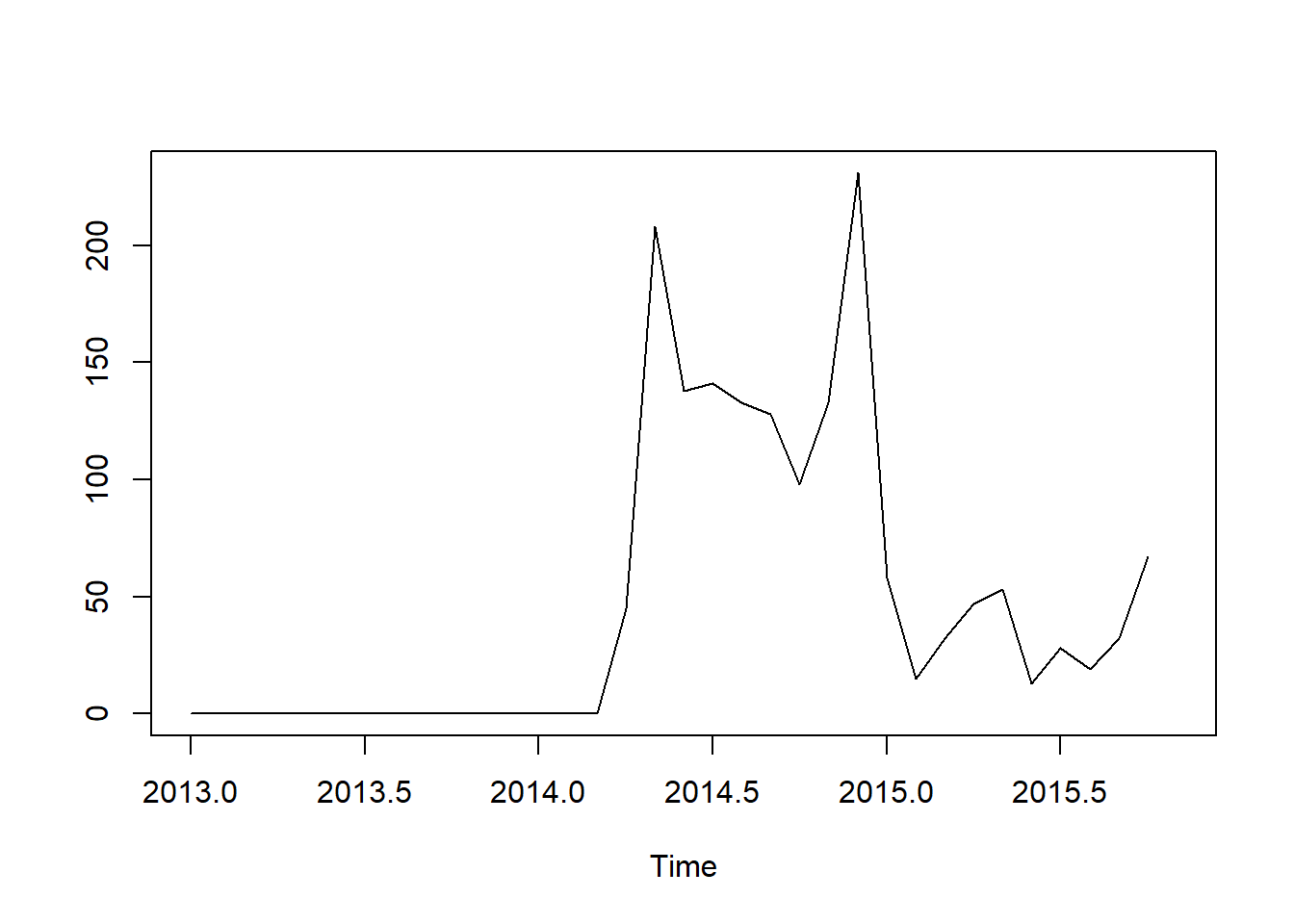pred112$pred ## Nov ## 2015 50.25803 fit011 <- arima(ts, order = c(0, 1, 1)) pred011 <- predict(fit011) ts.plot(ts, pred011$pred, lty = c(1,3))pred011$pred ## Nov ## 2015 55.73907 Exciting! We see sales increasing slightly in the past few months in this subset after a dip, and the model accounts for that and estimates 50 items sold for the next month in the cycle.. I personal think the (1, 1, 2) ARIMA seems to fit better as a generalization if I’m looking for one to fit all possible shop-item combo’s. Speaking of, I need to write up a function to run through the store item combos and fit the model/report it to a list.. I’ll finally take the list and reorder it to the sample submission and test the fits on kaggle. get_preds <- function(){ #get store id and item id from test data preds <-list() for(i in 1:nrow(test)){ store_id <- test[i,2] items_id <- test[i, 3] ts_test <- subset(ts_train, ts_train$shop_id == store_id & ts_train$item_id == items_id) ts <- rnorm(34, m = 0, sd = 0) ts <- ts(ts, start = 2013, frequency = 12) for (k in 0:33){ if(k %in% ts_test$date_block_num){
j <- which(ts_test$date_block_num == k) num <- ts_test[j,4] ts[k+1] <- as.numeric(num) } } if (max(ts) <= 0){ preds[i] <- 0 } else{ #try(fit <- arima(ts, order = c(1, 1, 2), method = "ML")) ##CHANGE THIS TO CHANGE MODEL fit <- HoltWinters(ts, alpha = .35, beta = FALSE, gamma = FALSE) pred <- predict(fit) preds[i] <- pred } if (i %% 1000 == 0){ print(i) } } } #get_preds() Alright, we should have a list of predictions from the ARIMA model.. Lets check a few then move them to their proper column in the sample submission from KAggle. NOTE: I came back to this after the first few submissions and gave exponential smoothing a try.. SO while my ARIMA is commented out, this was the primaryt exploration I performed.Exponential smoothing was slighltly better than ARIMA- (0.1 decrease in root mean square error)! #clipper<- function(preds, UB=11, LB=0) pmax( LB, pmin( preds, UB)) #clipped_preds <- clipper(preds) #print(preds) #print(preds) #print(preds) #for (i in 1:nrow(sample_sub)){ # sample_sub[i,2] <- clipped_preds[i] #} #sample_sub$item_cnt_month <- round(sample_sub\$item_cnt_month)
#sample_sub[1:10,]

All thats left is to write it out for submission:

#write.csv(sample_sub, file = "D://projects/kaggle/time_series/exp_smooth_11_clip.csv", row.names = FALSE)

## Kaggle Results

Top 28% of contestants (146/531)!!. I’m happy with it for my first time running a time series model. This model may also be running into trouble due to over-generalizing the auto-regressive and moving average values for each shop-item combo.. It’s definately not the strongest for each individual combination. I think that is at least partially the reasin the exponential smoothing method performs more accurately for the data.

Regardless, I’m happy with my first epxlorations into time-series analysis in R. A root mean squared error of only 1.06 is pretty decent for predicting how many items a store will sell based on only 34 months of data. Including some more details about the items (like category or price) could possibly enhance this even more I’m sure.

As my goal here was to fit and test a time-series model though, I’d say this is successful.